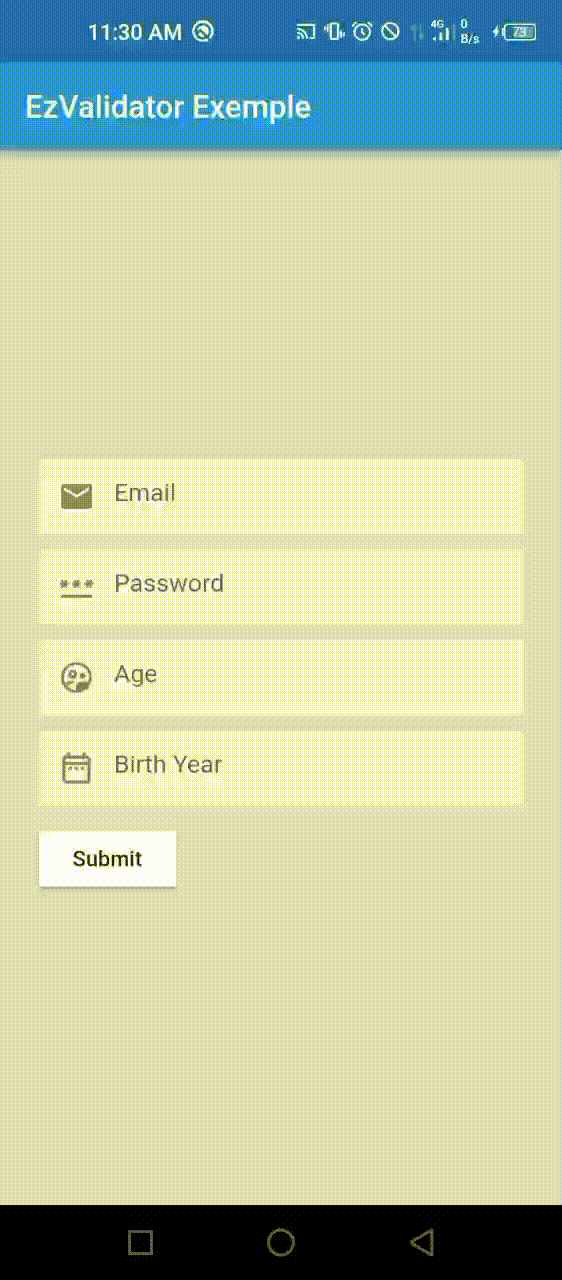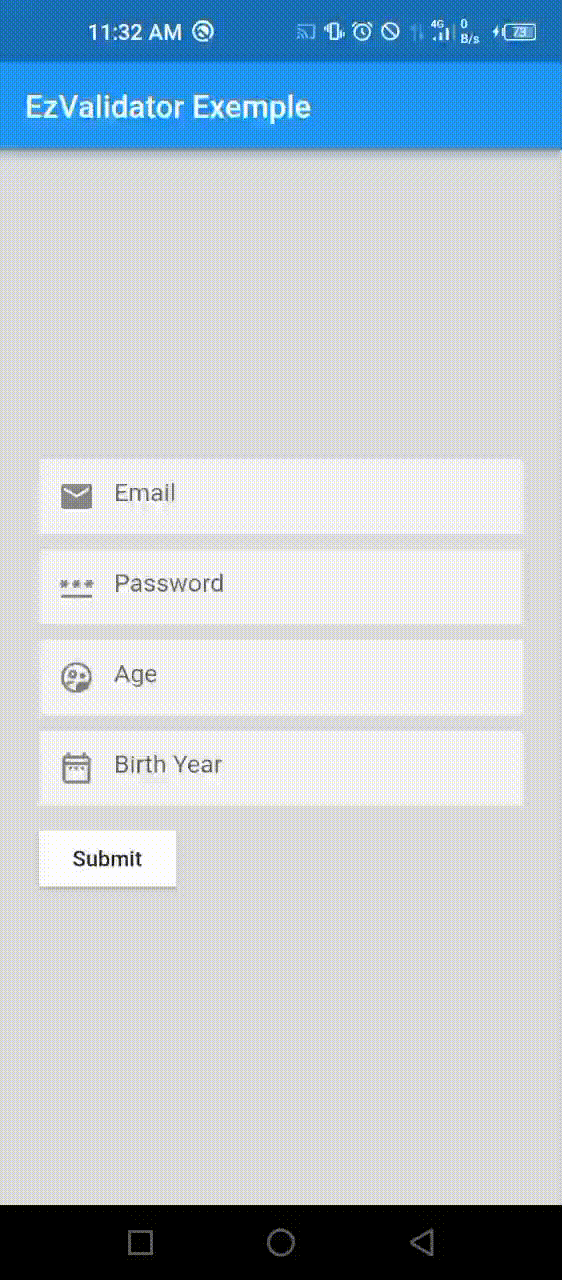# ez_validator

Dead simple field/object schema validation for flutter
EzValidator api is inspired by Yup

## EzValidator

EzValidator is a flutter schema builder for value validation. Define a schema, validate the shape of an existing value, or both. EzValidator allow single or object validations

## Installing

``````dependencies:
``````

## Usage

You define your schema object. check the exemple folder of how to use validation with multiple form with showing errors

``````EzSchema mySchema = EzSchema.shape({
"email": EzValidator().required().email().build(),
.required()
.minLength(6)
.matches(
r'^(?=.*[A-Za-z])(?=.*\d)(?=.*[@\$!%*#?&])[A-Za-z\d@\$!%*#?&]{6,}\$',
'Minimum six characters, at least one letter, one number and one special character')
.build(),
"age": EzValidator().required().min(18).build(),
}, identicalKeys: true);

// check validity

Map<String, String> errors = mySchema.validateSync({
"email": 'iheb@pixelium.tn',
"age": "17"
});

print(errors);

// output if the form not valid
//
//  {
//    "password": "Minimum six characters, at least one letter, one number and one special character",
//    "age": "The field must be greater than or equal to 18"
//  }
//
//
// output if the form is valid
//{}

``````

exemple of using defaultTest

``````EzSchema mySchema = EzSchema.shape({
.....
"sum": EzValidator()
.required()
.defaultTest(
'Test not valid please recheck', (v) => int.parse(v as String) > 25)
.build(),
....
};

``````

## Showcase

with default locale with custom locale## Methods

### `.required([String? message])`

Validates if the value is not empty or null.

### `.minLength(int minLength, [String? message])`

Validates if the value length is not less than `minLength`.

### `.maxLength(int maxLength, [String? message])`

Validates if the value length is not more than `maxLength`.

### `.min(int min, [String? message])`

Validates if the value is lesser or equal to `min`.

### `.max(int max, [String? message])`

Validates if the value is higher or equal `max`.

### `.positive([String? message])`

Validates if the value is positive.

### `.negative([String? message])`

Validates if the value is negative.

### `.email([String? message])`

Checks if the value is an email address.

### `.uiid([String? message])`

Checks if the value is a valid uuid.

### `.number([String? message])`

Checks if the value is a number.

### `.boolean([String? message])`

Checks if the value is boolean (true,false).

### `.date([String? message])`

Checks if the value is a date type.

### `.notNumber([String? message])`

Checks if the value is not a number.

### `.lowerCase([String? message])`

Checks if the value is on lowercase.

### `.upperCase([String? message])`

Checks if the value is on uppercase.

### `.oneOf(List<String?> items,[String? message])`

Checks if the value is one of the follwoing `items`.

### `.notOneOf(List<String?> items,[String? message])`

Checks if the value is not one of the follwoing `items`.

### `.phone([String? message])`

Checks if the value is a phone number.

### `.ip([String? message])`

Checks if the value is correct IPv4 address.

### `.ipv6([String? message])`

Checks if the value is correct IPv6 address.

### `.url([String? message])`

Checks if the value is correct url address.

### `.matches(String pattern, [String? message])`

Validates if the value does matches with the pattern.

### `.defaultTest(String message, bool Function(String? v) test)`

Validates the value with your own validation logic

Validator code inspired from form_validator